Learn how to implement adversarial validation that builds a classifier to determine if your data is from the training or testing sets. If you can do this, then your data has issues, and your adversarial validation model can help you diagnose the problem.

If you were to study some of the competition-winning solutions on Kaggle, you might notice references to “adversarial validation” (like this one). What is it?

In short, we build a classifier to try to predict which data rows are from the training set, and which are from the test set. If the two datasets came from the same distribution, this should be impossible. But if there are systematic differences in the feature values of your training and test datasets, then a classifier will be able to successfully learn to distinguish between them. The better a model you can learn to distinguish them, the bigger the problem you have.

But the good news is that you can analyze the learned model to help you diagnose the problem. And once you understand the problem, you can go about fixing it.

This post is meant to accompany a YouTube video I made to explain the intuition of Adversarial Validation. This blog post walks through the code implementation of the example presented in this video but is complete enough to be self-contained. You can find the complete code for this post on GitHub.

### Learning the Adversarial Validation model

First, some boilerplate import statements to avoid confusion:

```import pandas as pd
from catboost import Pool, CatBoostClassifier
```

### Data Preparation

For this tutorial, we're going to be using the IEEE-CIS Credit Card Fraud Detection dataset from Kaggle. First, I'll assume you've loaded the training and test data into pandas DataFrames and called them df_train and df_test, respectively. Then we'll do some basic cleaning by replacing missing values.

```# Replace missing categoricals with ""
df_train.loc[:,cat_cols] = df_train[cat_cols].fillna('')
df_test.loc[:,cat_cols] = df_test[cat_cols].fillna('')

# Replace missing numeric with -999
df_train = df_train.fillna(-999)
df_test = df_test.fillna(-999)

```

For adversarial validation, we want to learn a model that predicts which rows are in the training dataset, and which are in the test set. We, therefore, create a new target column in which the test samples are labeled with 1 and the train samples with 0, like this:

```df_train['dataset_label'] = 0
df_test['dataset_label'] = 1
target = 'dataset_label'

```

This is the target that we'll train a model to predict. Right now, the train and test datasets are separate, and each dataset has only one label for the target value. If we trained a model on this training set, it would just learn that everything was 0. We want to instead shuffle the train and test datasets, and then create new datasets for fitting and evaluating the adversarial validation model. I define a function for combining, shuffling, and re-splitting:

```def create_adversarial_data(df_train, df_test, cols, N_val=50000):
df_master = pd.concat([df_train[cols], df_test[cols]], axis=0)

features = cat_cols + numeric_cols + ['TransactionDT']
all_cols = features + [target]

```

The new datasets, adversarial_train and adversarial_test, include a mix of the original training and test sets, and the target indicates the original dataset. Note: I added TransactionDT to the feature list. The reason for this will become apparent.

For modeling, I'm going to be using Catboost. I finish data preparation by putting the DataFrames into Catboost Pool objects.

```train_data = Pool(
cat_features=cat_cols
)
holdout_data = Pool(
cat_features=cat_cols
)

```

### Modeling

This part is simple: we just instantiate a Catboost Classifier and fit it on our data:

```params = {
'iterations': 100,
'eval_metric': 'AUC',
'od_type': 'Iter',
'od_wait': 50,
}

model = CatBoostClassifier(**params)
_ = model.fit(train_data, eval_set=holdout_data)

```

Let's go ahead and plot the ROC curve on the holdout dataset: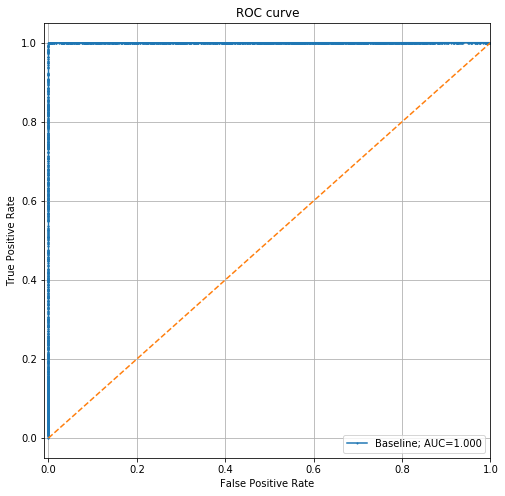This is a perfect model, which means there's a clear way to tell whether any given record is in the training or test sets. This is a violation of the assumption that our training and test sets are identically distributed.

### Diagnosing the problem and iterating

To understand how the model was able to do this, let's look at the most important features: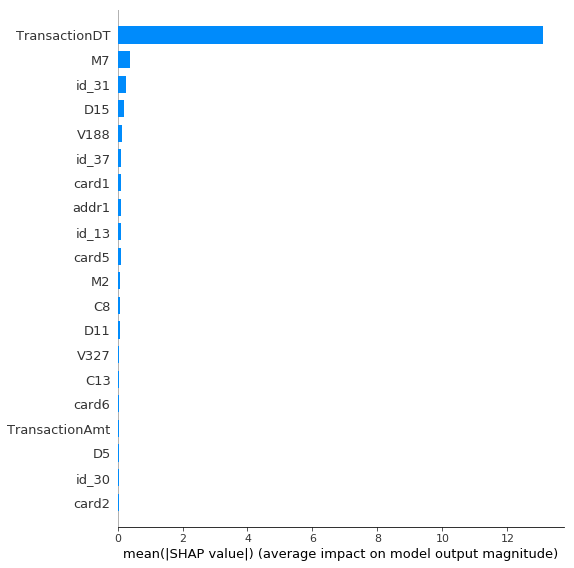The TransactionDT is by far the most important feature. And that makes total sense given that the original training and test datasets came from different periods (the test set occurs in the future of the training set). The model has just learned that if the TransactionDT is larger than the last training sample, it's in the test set.

I included the TransactionDT just to make this point–it's not advised to throw a raw date in as a model feature normally. But it's good news that this technique found it in such a dramatic fashion. This analysis would clearly help you identify such an error.

Let's eliminate TransactionDT, and run this analysis again.

```params2 = dict(params)
params2.update({"ignored_features": ['TransactionDT']})
model2 = CatBoostClassifier(**params2)
_ = model2.fit(train_data, eval_set=holdout_data)

```

Now the ROC curve looks like this: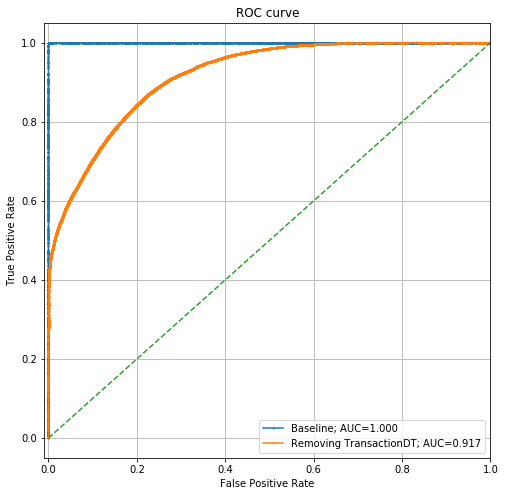It's still a fairly strong model with AUC > 0.91, but much weaker than before. Let's look at the feature importances for this model: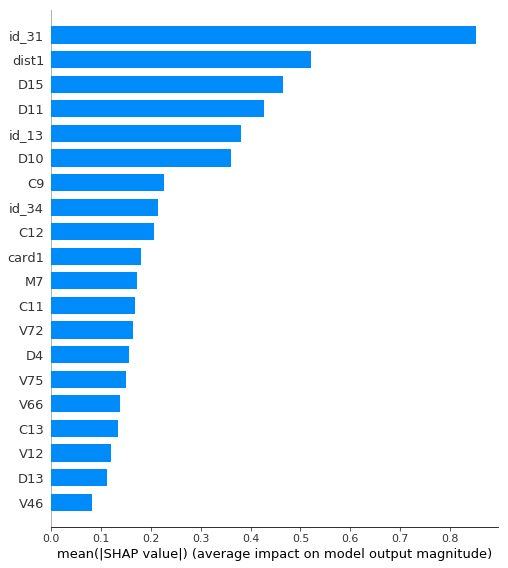Now, id_31 is the most important feature. Let's look at some values to understand what it is.

```[
'', 'samsung browser 6.2', 'mobile safari 11.0',
'chrome 62.0', 'chrome 62.0 for android', 'edge 15.0',
'mobile safari generic', 'chrome 49.0', 'chrome 61.0', 'edge 16.0'
]

```

This column contains software version numbers. Clearly, this is similar in concept to including a raw date, because the first occurrence of a particular software version will correspond to its release date.

Let's get around this problem by dropping any characters that are not letters from the column:

```def remove_numbers(df_train, df_test, feature):
df_train.loc[:, feature] = df_train[feature].str.replace(r'[^A-Za-z]', '', regex=True)
df_test.loc[:, feature] = df_test[feature].str.replace(r'[^A-Za-z]', '', regex=True)

remove_numbers(df_train, df_test, 'id_31')

```

Now the values of our column look like this:

```[
'UNK', 'samsungbrowser', 'mobilesafari',
'chrome', 'chromeforandroid', 'edge',
'mobilesafarigeneric', 'safarigeneric',
]

```

Let's train a new adversarial validation model using this cleaned column:

```adversarial_train_scrub, adversarial_test_scrub = create_adversarial_data(
df_train,
df_test,
all_cols,
)

train_data_scrub = Pool(
cat_features=cat_colsc
)

holdout_data_scrub = Pool(
cat_features=cat_colsc
)

model_scrub = CatBoostClassifier(**params2)
_ = model_scrub.fit(train_data_scrub, eval_set=holdout_data_scrub)

```

The ROC plot now looks like this: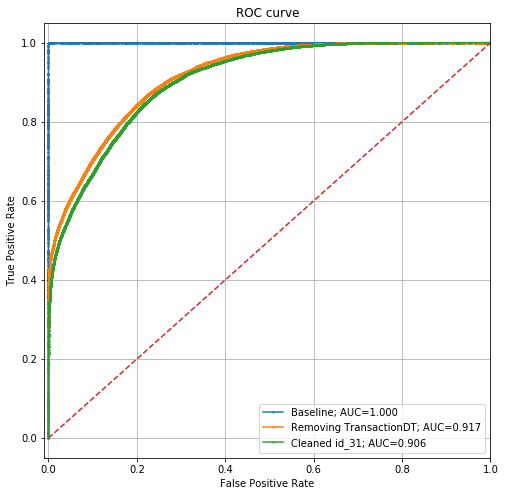The performance has dropped from an AUC of 0.917 to 0.906. This means that we've made it a little harder for a model to distinguish between our training and test datasets, but it's still quite capable.

### Conclusion

When we naively tossed the transaction date into the feature set, the adversarial validation process helped to clearly diagnose the problem. Additional iterations gave us more clues that a column containing software version information had clear differences between the training and test sets.

But what the process is not able to do is tell us how to fix it. We still need to apply our creativity here. In this example, we simply removed all numbers from the software version information, but this is throwing away potentially useful information and might ultimately hurt our fraud modeling task, which is our real goal. The idea is that you want to remove information that is not important for predicting fraud but is important for separating your training and test sets.

A better approach might have been to find a dataset that gave the software release dates for each software version, and then created a “days since release” column that replaced the raw version number. This might make for a better match for the train and test distributions while also maintaining the predictive power that software version information encodes.

Related: